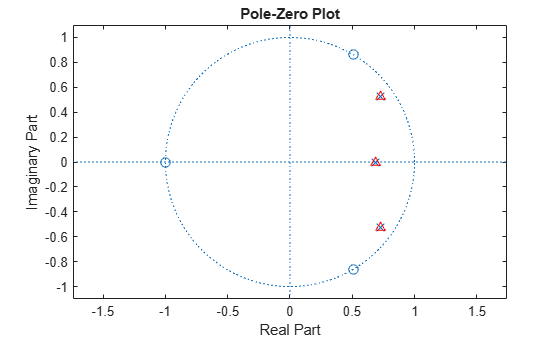residuez

Z-transform partial-fraction expansion

Description

example

[ro,po,ko] = residuez(bi,ai) finds the residues, poles, and direct terms of a partial fraction expansion of the ratio of numerator and denominator polynomials, b and a.

[bo,ao] = residuez(ri,pi,ki) with three input arguments and two output arguments, converts the partial fraction expansion back to polynomials with coefficients in row vectors b and a.

Examples

collapse all

Compute the partial-fraction expansion corresponding to the third-order IIR lowpass filter described by the transfer function

$H\left(z\right)=\frac{0.05634\left(1+{z}^{-1}\right)\left(1-1.0166{z}^{-1}+{z}^{-2}\right)}{\left(1-0.683{z}^{-1}\right)\left(1-1.4461{z}^{-1}+0.7957{z}^{-2}\right)}.$

Express the numerator and denominator as polynomial convolutions.

b0 = 0.05634;
b1 = [1  1];
b2 = [1 -1.0166 1];
a1 = [1 -0.683];
a2 = [1 -1.4461 0.7957];

b = b0*conv(b1,b2);
a = conv(a1,a2);

Compute the residues, poles, and direct terms of the partial-fraction expansion.

[r,p,k] = residuez(b,a)
r = 3×1 complex

-0.1153 - 0.0182i
-0.1153 + 0.0182i
0.3905 + 0.0000i

p = 3×1 complex

0.7230 + 0.5224i
0.7230 - 0.5224i
0.6830 + 0.0000i

k = -0.1037

Plot the poles and zeros of the transfer function and overlay the poles you just found.

zplane(b,a)
hold on
plot(p,'^r')
hold offUse residuez again to reconstruct the transfer function.

[bn,an] = residuez(r,p,k)
bn = 1×4

0.0563   -0.0009   -0.0009    0.0563

an = 1×4

1.0000   -2.1291    1.7834   -0.5435

Input Arguments

collapse all

Polynomial coefficients, specified as vectors. Vectors b and a specify the coefficients of the polynomials of the discrete-time system b(z)/a(z) in descending powers of z.

$\begin{array}{c}B\left(z\right)={b}_{0}+{b}_{1}{z}^{-1}+{b}_{2}{z}^{-2}+\cdots +{b}_{m}{z}^{-m}\\ A\left(z\right)={a}_{0}+{a}_{1}{z}^{-1}+{a}_{2}{z}^{-2}+\cdots +{a}_{n}{z}^{-n}\end{array}$

If there are multiple roots and a > n-1,

$\frac{B\left(z\right)}{A\left(z\right)}=\frac{r\left(1\right)}{1-p\left(1\right){z}^{-1}}+\cdots +\frac{r\left(n\right)}{1-p\left(n\right){z}^{-1}}+k\left(1\right)+k\left(2\right){z}^{-1}+\cdots +k\left(m-n+1\right){z}^{-\left(m-n\right)}$

Residues of the partial fraction, specified as a vector.

Poles of the partial fraction, specified as a vector.

Direct terms, specified as a row vector.

Output Arguments

collapse all

Residues of the partial fraction, returned as a vector.

Pole of the partial fraction, returned as a vector. The number of poles is

n = length(a)-1 = length(r) = length(p)

If p(j) = ... = p(j+s-1) is a pole of multiplicity s, then the expansion includes terms of the form

$\frac{r\left(j\right)}{1-p\left(j\right){z}^{-1}}+\frac{r\left(j+1\right)}{{\left(1-p\left(j\right){z}^{-1}\right)}^{2}}+\cdots +\frac{r\left(j+{s}_{r}-1\right)}{{\left(1-p\left(j\right){z}^{-1}\right)}^{s}}$

Direct terms, returned as a row vector. The direct term coefficient vector k is empty if length(b) is less than length(a); otherwise:

length(k) = length(b) - length(a) + 1

Polynomial coefficients, returned as vectors.

Algorithms

residuez converts a discrete time system, expressed as the ratio of two polynomials, to partial fraction expansion, or residue, form. It also converts the partial fraction expansion back to the original polynomial coefficients.

Note

Numerically, the partial fraction expansion of a ratio of polynomials is an ill-posed problem. If the denominator polynomial is near a polynomial with multiple roots, then small changes in the data, including round-off errors, can cause arbitrarily large changes in the resulting poles and residues. You should use state-space or pole-zero representations instead.

residuez applies standard MATLAB® functions and partial fraction techniques to find r, p, and k from b and a. It finds

• The direct terms a using deconv (polynomial long division) when length(b) > length(a)-1.

• The poles using p = roots(a).

• Any repeated poles, reordering the poles according to their multiplicities.

• The residue for each nonrepeating pole pj by multiplying b(z)/a(z) by 1/(1 - pjz−1) and evaluating the resulting rational function at z = pj.

• The residues for the repeated poles by solving

S2*r2 = h - S1*r1

for r2 using \. h is the impulse response of the reduced b(z)/a(z), S1 is a matrix whose columns are impulse responses of the first-order systems made up of the nonrepeating roots, and r1 is a column containing the residues for the nonrepeating roots. Each column of matrix S2 is an impulse response. For each root pj of multiplicity sj, S2 contains sj columns representing the impulse responses of each of the following systems.

$\frac{1}{1-{p}_{j}{z}^{-1}},\frac{1}{{\left(1-{p}_{j}{z}^{-1}\right)}^{2}},\cdots ,\frac{1}{{\left(1-{p}_{j}{z}^{-1}\right)}^{{s}_{j}}}$

The vector h and matrices S1 and S2 have n + xtra rows, where n is the total number of roots and the internal parameter xtra, set to 1 by default, determines the degree of over-determination of the system of equations.

Note

The residue function in the standard MATLAB language is very similar to residuez. It computes the partial fraction expansion of continuous-time systems in the Laplace domain (see reference ), rather than discrete-time systems in the z-domain as does residuez.

 Oppenheim, Alan V., Ronald W. Schafer, and John R. Buck. Discrete-Time Signal Processing. 2nd Ed. Upper Saddle River, NJ: Prentice Hall, 1999.